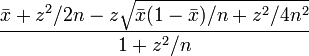# Wilson score interval test

Enter a number of votes for each value:
 1 star 2 stars 3 stars 4 stars 5 stars
Results:
ItemValueDescription
Total number of votes3This value will be seen by users in the problem page.
Average value3This value will be seen by users in the problem page.
Score (lower bound)1.5013258119938This value will be used to order problems in highest rated list.
Score (upper bound)4.4986741880062(not used)

The score is calculated by Wilson score confidence interval (http://www.evanmiller.org/how-not-to-sort-by-average-rating.html) with 95% of confidence:.
where x-bar is ((the average number of stars) - 1) / 4, and the final score is 1 + 4 * (the result of the formula).

The old formula was used until Apr 30, 2012. Thanks to Margus for the correction and logical explanation!